• //哈夫曼树算法 #include using namespace std; const int n=5; const int m=2*n-1; const int float_max=20; typedef int datatype; typedef struct { float weight; //定义权重 int parent; //定义双亲在...
//哈夫曼树算法
#include<iostream>
using namespace std;
const int n=5;
const int m=2*n-1;
const int float_max=20;
typedef int datatype;
typedef struct
{
float weight;			//定义权重
int parent;				//定义双亲在向量中的下标
int lchild,rchild;		//定义左右子树
}	nodetype;				//结点类型
typedef nodetype hftree[m]; //哈夫曼树类型，数组从0号单元开始使用
hftree T;					//哈夫曼树向量

//哈夫曼树的构造
void huffman(hftree T)
{
int i,j,p1,p2;
float small1,small2;
for(i=0;i<n;i++)        //初始化
{
T[i].parent=-1;		//无双亲，即为根结点，尚未合并过
T[i].lchild=T[i].rchild=-1;//左右孩子指针置为-1
}
for(i=0;i<n;i++)
{
cin>>T[i].weight;   //输入n个叶子的权
}
for(i=n;i<m;i++)		//进行n-1次合并，产生n-1个新结点
{
p1=p2=-1;
small1=small2=float_max;	//float_max为float类型的最大值
for(j=0;j<=i-1;j++)
{
if(T[j].parent!=-1) continue;//不考虑已合并过的点
if(T[j].weight<small1)			//修改最小权和次小权及位置
{
small2=small1;
small1=T[j].weight;
p2=p1;
p1=j;
}
else if(T[j].weight<small2)		//修改次小权及位置
{
small2=T[j].weight;
p2=j;
}
}
T[p1].parent=T[p2].parent=i;		//新根
T[i].parent=-1;
T[i].lchild=p1;
T[i].rchild=p2;
T[i].weight=small1+small2;			//新结点的权值为最小权与次小权之和
}
}

int main()
{
hftree T;
cout<<"          欢迎测试！！！！   "<<endl;
cout<<"----------测试开始-----------"<<endl;
cout<<"首先调用哈夫曼树构造的函数：huffman"<<endl;
cout<<"按下标顺序输入叶子的权重:"<<endl;
cout<<" 0  1  2  3  4  5  6  7  8 "<<endl;
huffman(T);
cout<<"输出合并后的哈夫曼树的所有结点："<<endl;
cout<<" 0  1  2  3  4  5  6  7  8 "<<endl;
for(int i=0;i<m;i++)
{
cout<<T[i].weight<<"  ";
}
system("pause");
return 0;
}
展开全文数据结构 c++ float 测试
• 先构建以这个n个结点为叶子结点的哈夫曼树，然后由哈夫曼树产生各叶子结点对应字符的哈夫曼编码。 (0）哈夫曼树：给定n个权值作为n个叶子结点，构造一棵二叉树，若树的带权路径长度达到最小，则这棵树被称为哈夫曼树...
哈夫曼编码
一、【问题描述】
设要编码的字符集为{d1,d2,…,dn},它们出现的频率为{w1，w2，…,wn}，应用哈夫曼树构造最优的不等长的由0，1构成的编码方案。
二、【问题求解】
先构建以这个n个结点为叶子结点的哈夫曼树，然后由哈夫曼树产生各叶子结点对应字符的哈夫曼编码。
(0）哈夫曼树：给定n个权值作为n个叶子结点，构造一棵二叉树，若树的带权路径长度达到最小，则这棵树被称为哈夫曼树。
(1) 路径和路径长度：在一棵树中，从一个结点往下可以达到的孩子或孙子结点之间的通路，称为路径。通路中分支的数目称为路径长度。若规定根结点的层数为1，则从根结点到第L层结点的路径长度为L-1。
(2) 结点的权及带权路径长度：若将树中结点赋给一个有着某种含义的数值，则这个数值称为该结点的权。结点的带权路径长度为：从根结点到该结点之间的路径长度与该结点的权的乘积。
(3) 树的带权路径长度：树的带权路径长度规定为所有叶子结点的带权路径长度之和，记为WPL。
⭐对于一些基本概念，此处不进行更多赘述
如果还有疑惑可以参考这篇博文：
传送门→_→   哈夫曼树+哈夫曼编码
构造一棵哈夫曼树的方法如下：
①由给定的n个权值， n个权值分别设为 w1、w2、…、wn，构造n棵只有1个叶子结点的二叉树，从而得到一个二叉树的集合F={T1,T2,…Tn}。
②在F中选取根节点的权值最小和次小的两颗二叉树作为左、右子树构造一棵新的二叉树，这颗新的二叉树根节点的权值为其左、右子树根节点权值之和。即合并两棵二叉树为一棵二叉树。
③重复步骤②，当F中只剩下一棵二叉树时，这棵二叉树便是所要建立的哈夫曼树。
例如给定a~d四个字符，它们的权值集合为w={100,10,50,20}
首先构造出哈夫曼树，过程如下图：接下来对字符进行编码并求出WPL三、【代码实现】
如下：
#pragma warning(disable:4786) //用于屏蔽标识符过长导致的warning
#include <iostream>
#include <queue>
#include <vector>
#include <string>
#include <map>
using namespace std;
#define MAX 101
int n;
struct HTreeNode				//哈夫曼树结点类型
{
char data;					//字符
int weight;					//权值
int parent;					//双亲的位置
int lchild;					//左孩子的位置
int rchild;					//右孩子的位置
};
HTreeNode ht[MAX];				//哈夫曼树
map<char,string> htcode;			//哈夫曼编码

struct NodeType		//优先队列结点类型
{
int no;				//对应哈夫曼树ht中的位置
char data;			//字符
int  weight;		//权值
bool operator<(const NodeType &s) const
{					//运算符重载进行从小到大的递增排序
return s.weight<weight;
}
};
void CreateHTree()						//构造哈夫曼树
{
NodeType e,e1,e2;
priority_queue<NodeType> qu;
for (int k=0;k<2*n-1;k++)	//设置所有结点的指针域
ht[k].lchild=ht[k].rchild=ht[k].parent=-1;
for (int i=0;i<n;i++)				//将n个结点进队qu
{
e.no=i;
e.data=ht[i].data;
e.weight=ht[i].weight;
qu.push(e);
}
for (int j=n;j<2*n-1;j++)			//构造哈夫曼树的n-1个非叶结点
{
e1=qu.top();  qu.pop();		//出队权值最小的结点e1
e2=qu.top();  qu.pop();		//出队权值次小的结点e2
ht[j].weight=e1.weight+e2.weight; //构造哈夫曼树的非叶结点j
ht[j].lchild=e1.no;
ht[j].rchild=e2.no;
ht[e1.no].parent=j;			//修改e1.no的双亲为结点j
ht[e2.no].parent=j;			//修改e2.no的双亲为结点j
e.no=j;						//构造队列结点e
e.weight=e1.weight+e2.weight;
qu.push(e);
}
}
void CreateHCode()			//构造哈夫曼编码
{
string code;
code.reserve(MAX);
for (int i=0;i<n;i++)	//构造叶结点i的哈夫曼编码
{
code="";
int curno=i;
int f=ht[curno].parent;
while (f!=-1)				//循环到根结点
{
if (ht[f].lchild==curno)	//curno为双亲f的左孩子
code='0'+code;
else					//curno为双亲f的右孩子
code='1'+code;
curno=f; f=ht[curno].parent;
}
htcode[ht[i].data]=code;	//得到ht[i].data字符的哈夫曼编码
}
}
void DispHCode()					//输出哈夫曼编码
{
map<char,string>::iterator it;
for (it=htcode.begin();it!=htcode.end();++it)
cout << "    " << it->first << ": " << it->second <<	endl;
}
void DispHTree()					//输出哈夫曼树
{
for (int i=0;i<2*n-1;i++)
{
printf("    data=%c, weight=%d, lchild=%d, rchild=%d, parent=%d\n",
ht[i].data,ht[i].weight,ht[i].lchild,ht[i].rchild,ht[i].parent);
}
}
int WPL()				//求WPL
{
int wps=0;
for (int i=0;i<n;i++)
wps+=ht[i].weight*htcode[ht[i].data].size();
return wps;
}

int main()
{
n=4;
ht.data='a'; ht.weight=100;		//置初值即n个叶子结点
ht.data='b'; ht.weight=10;
ht.data='c'; ht.weight=50;
ht.data='d'; ht.weight=20;
CreateHTree();					//建立哈夫曼树
printf("构造的哈夫曼树:\n");
DispHTree();
CreateHCode();					//求哈夫曼编码
printf("产生的哈夫曼编码如下:\n");
DispHCode();					//输出哈夫曼编码
printf("WPL=%d\n",WPL());
return 0;
}

代码运行截图：本文参考自《算法设计与分析》李春葆第二版


展开全文队列 数据结构 c语言
• 一、哈夫曼树的基本概念在一棵二叉树中，定义从A结点到B结点所经过的分支序列叫做从A结点到B结点的路径。从A结点到B结点所经过的分支个数叫做从A结点到B结点的路径长度从二叉树的根结点到二叉树中所有叶结点的路径...
一、哈夫曼树的基本概念在一棵二叉树中，定义从A结点到B结点所经过的分支序列叫做从A结点到B结点的路径。从A结点到B结点所经过的分支个数叫做从A结点到B结点的路径长度从二叉树的根结点到二叉树中所有叶结点的路径长度之和称作该二叉树的路径长度。如果二叉树中的叶结点都带有权值，我们可以把这个定义加以推广。设二叉树有n个带权值的叶结点，定义从二叉树的根结点到二叉树中所有叶结点的路径长度与相应叶结点权值的乘积之和为该二叉树的带权路径长度（WPL），即：WPL =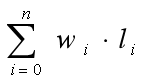wi为第i个叶结点的权值，li为从根结点到第i个叶结点的路径长度。二、哈夫曼树具有相同叶结点和不同带权路径长度的二叉树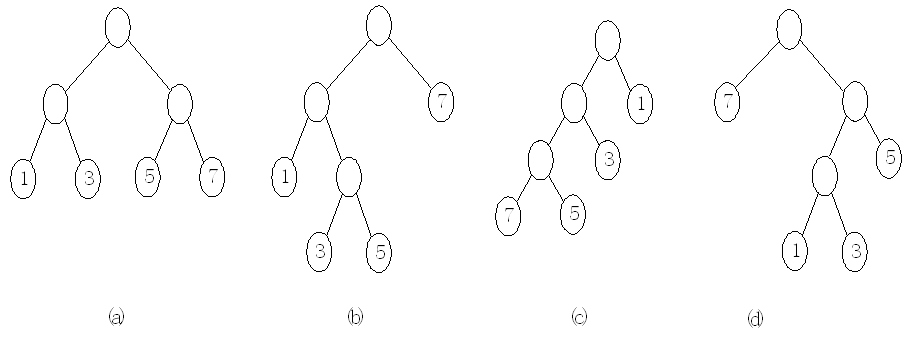（a）WPL = 1×2+3×2+5×2+7×2 = 32（b）WPL = 1×2+3×3+5×3+7×1 = 33（c）WPL = 7×3+5×3+3×2+1×1 = 43（d）WPL = 1×3+3×3+5×2+7×1 = 29三、哈夫曼树构造算法根据哈夫曼树的定义，要使一棵二叉树的带权路径长度WPL值最小，必须使权值越大的叶结点越靠近根结点。哈夫曼提出的构造哈夫曼树构造算法为：（1）由给定的n个权值{w1,w2,…,wn}构造n棵只有根 结点的二叉树，从而得到一个二叉树森林F={T1,T2,…,Tn}。（2）在二叉树森林F中选取根结点的权值最小和次小的两棵二叉树作为新的二叉树的左右子树构造新的二叉树，新的二叉树的根结点权值为左右子树根结点权值之和。（3）在二叉树森林F中删除作为新二叉树左右子树的两棵二叉树，将新二叉树加入到二叉树森林F中。（4）重复步骤（2）和（3），当二叉树森林F中只剩下一棵二叉树时，这棵二叉树就是所构造的哈夫曼树。对于一组给定的叶结点，设它们的权值集合为{7,5,3,1}，按哈夫曼树构造算法对此集合构造哈夫曼树的过程如图所示。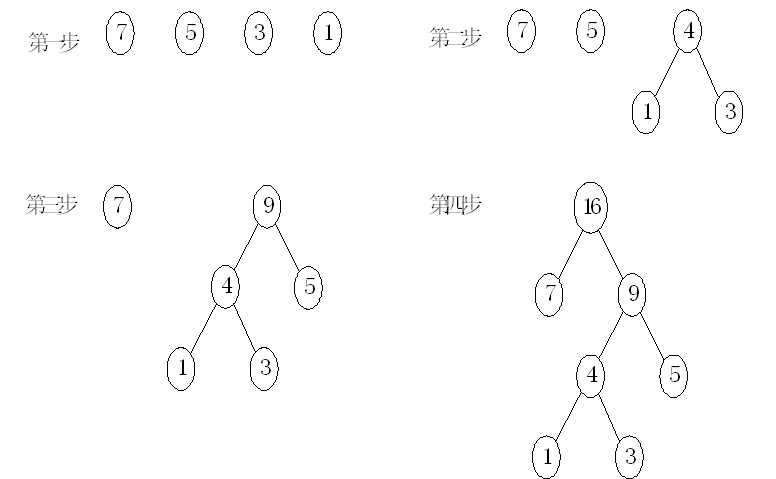四、哈夫曼树编码问题哈夫曼树可用于构造代码总长度最短的编码方案。具体构造方法如下：设需要编码的字符集合为{d1,d2,…,dn}，各个字符在电文中出现的次数集合为{w1,w2,…,wn}，以d1,d2,…,dn作为叶结点，以w1,w2,…,wn作为各叶结点的权值构造一棵二叉树，规定哈夫曼树中的左分支为0，右分支为1，则从根结点到每个叶结点所经过的分支对应的0和1组成的序列便为该结点对应字符的编码。这样的代码总长度最短的不等长编码称之为哈夫曼编码。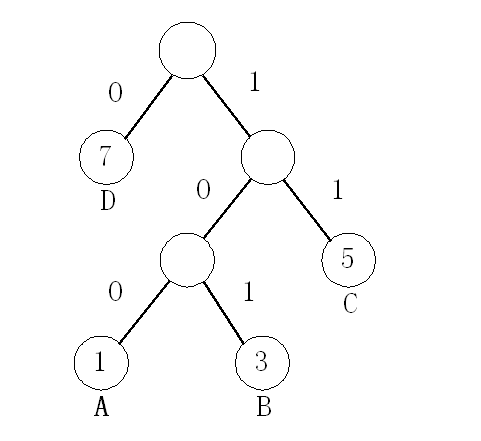哈夫曼编码的数据结构设计设计哈夫曼树的结点存储结构为双亲孩子存储结构。存放哈夫曼编码的存储结构为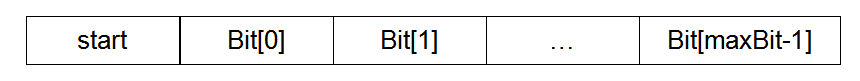五、哈夫曼树以及哈夫曼编码的实现//哈夫曼树的结点类
public class HaffNode {

int weight; //权值
int parent;//双亲
int flag;//标志
int leftChild; //左孩子
int rightChild;//右孩子

HaffNode() {

}

}//哈夫曼编码类
public class Code {

int[] bit;  //编码数组
int start;  //编码的开始下标
int weight; //权值

Code(int n) {
bit = new int[n];
start = n - 1;
}
}/哈夫曼树类
public class HaffmanTree {
//最大权值
static final int MAXVALUE = 1000;
int nodeNum; //叶子结点个数

public HaffmanTree(int n) {
this.nodeNum = n;
}

//构造哈夫曼树算法
public void haffman(int[] weight, HaffNode[] nodes) {
int n = this.nodeNum;
//m1,m2,表示最小的两个权值，x1,x2,表示最小两个权值对应的编号
int m1, m2, x1, x2;

//初始化所有的结点，对应有n个叶子结点的哈夫曼树，有2n-1个结点。
for (int i = 0; i < 2 * n - 1; i++) {
HaffNode temp = new HaffNode();
//初始化n个叶子结点
if (i < n) {
temp.weight = weight[i];
} else {
temp.weight = 0;
}
temp.parent = 0;
temp.flag = 0;
temp.leftChild = -1;
temp.rightChild = -1;
nodes[i] = temp;
}

//初始化n-1个非叶子结点
for (int i = 0; i < n - 1; i++) {
m1 = m2 = MAXVALUE;
x1 = x2 = 0;
for (int j = 0; j < n + i; j++) {
if (nodes[j].weight < m1 && nodes[j].flag == 0) {
m2 = m1;
x2 = x1;
m1 = nodes[j].weight;
x1 = j;
} else if (nodes[j].weight < m2 && nodes[j].flag == 0) {
m2 = nodes[j].weight;
x2 = j;
}
}
nodes[x1].parent = n + i;
nodes[x2].parent = n + i;
nodes[x1].flag = 1;
nodes[x2].flag = 1;
nodes[n + i].weight = nodes[x1].weight + nodes[x2].weight;
nodes[n + i].leftChild = x1;
nodes[n + i].rightChild = x2;
}

}

//哈弗曼编码算法
public void haffmanCode(HaffNode[] nodes, Code[] haffCode) {
int n = this.nodeNum;
Code code = new Code(n);
int child, parent;

for (int i = 0; i < n; i++) {
code.start = n - 1;
code.weight = nodes[i].weight;
child = i;
parent = nodes[child].parent;
while (parent != 0) {
if (nodes[parent].leftChild == child) {
code.bit[code.start] = 0;
} else {
code.bit[code.start] = 1;
}

code.start--;
child = parent;
parent = nodes[child].parent;
}

Code temp = new Code(n);
for (int j = code.start + 1; j < n; j++) {
temp.bit[j] = code.bit[j];
}
temp.weight = code.weight;
temp.start = code.start;
haffCode[i] = temp;
}

}
}public class Test {

public static void main(String[] args) {

int n = 4;
int[] weight = {1, 3, 5, 7};
HaffmanTree haffTree = new HaffmanTree(n);
HaffNode[] nodes = new HaffNode[2 * n - 1];
Code[] codes = new Code[n];
//构造哈夫曼树
haffTree.haffman(weight, nodes);
//生成哈夫曼编码
haffTree.haffmanCode(nodes, codes);

//打印哈夫曼编码
for (int i = 0; i < n; i++) {
System.out.print("Weight=" + codes[i].weight + " Code=");
for (int j = codes[i].start + 1; j < n; j++) {
System.out.print(codes[i].bit[j]);
}
System.out.println();
}
}

}运行结果：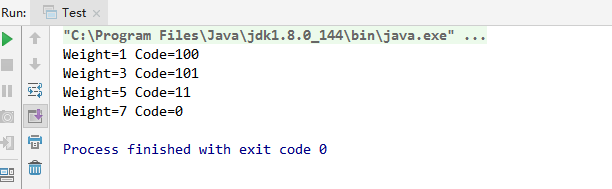展开全文• 经典哈夫曼树算法。Flash动态演示 哈夫曼编码是无损压缩当中最好的方法。它使用预先二进制描述来替换每个符号，长度由特殊符号出现的频率决定。常见的符号需要很少的位来表示，而不常见的符号需要很多为来表示。 ...
• 问题 C: 哈夫曼树 题目描述 哈夫曼树，第一行输入一个数n，表示叶结点的个数。需要用这些叶结点生成哈夫曼树，根据哈夫曼树的概念，这些结点有权值，即weight，题目需要输出所有结点的值与权值的乘积之和。 输入 ...
                                            问题 C: 哈夫曼树

题目描述

哈夫曼树，第一行输入一个数n，表示叶结点的个数。需要用这些叶结点生成哈夫曼树，根据哈夫曼树的概念，这些结点有权值，即weight，题目需要输出所有结点的值与权值的乘积之和。

输入

输入有多组数据。
每组第一行输入一个数n，接着输入n个叶节点（叶节点权值不超过100，2<=n<=1000）。

输出

输出权值。

样例输入

2
2 8
3
5 11 30

样例输出

10
62

AC代码：

#include<cstdio>
#include<queue>
using namespace std;
int main(){
int n;
long long temp,x,y;
while(scanf("%d",&n)!=EOF){
int ans = 0;
priority_queue<long long ,vector<long long>,greater<long long> >q;
for(int i = 0;i < n;i++){
scanf("%lld",&temp);
q.push(temp);
}
while(q.size() > 1){
x = q.top();
q.pop();
y = q.top();
q.pop();
q.push(x+y);
ans += x+y;
}
q.pop();
printf("%lld\n",ans);
}
return 0;
}
/**************************************************************
Problem: 1921
User: 2015212040209
Language: C++
Result: 正确
Time:0 ms
Memory:1172 kb
****************************************************************/


展开全文• 需要用这些叶结点生成哈夫曼树，根据哈夫曼树的概念，这些结点有权值，即weight，题目需要输出所有结点的值与权值的乘积之和。 输入 输入有多组数据。 每组第一行输入一个数n，接着输入n个叶节点（叶节点权值不超过...数据结构 c++ c语言
• 掌握哈夫曼树的构造算法。 掌握哈夫曼编码的构造算法。 【实验内容】 问题描述 输入一串字符,根据给定的字符串中字符出现的频率建立相应的哈夫曼树,构造哈夫曼编码表,在此基础上可以对压缩文件进行压缩(即编码),同时...
• 抽象的算法描述:将建立哈夫曼树、实现哈夫曼编码、哈夫曼译码都定义成 子函数的的形式, 然后在主函数中调用它们......数据结构课程设计设计题目: 哈夫曼树及其应用学 院:计算机科学与技术 专业:网络...用哈夫曼树...
• 本文实例讲述了C++数据结构与算法哈夫曼树的实现方法。分享给大家供大家参考，具体如下： 哈夫曼树又称最优二叉树，是一类带权路径长度最短的树。 对于最优二叉树，权值越大的结点越接近树的根结点，权值越小的...
• 算法描述如下： 1）将这N个结点分别作为N棵仅含一个结点的二叉树，构成森林F。 2）构造一个新结点，并从F中选取两棵根结点权值最小的作为新结点的左、右子，并且将新结点的权值 置为左、右子上根结点的权值...数据结构
• ## 哈夫曼树

千次阅读 2013-02-17 23:46:34
学习贪心算法的时候复习了一下哈夫曼树的构造，这里记录一下，参考链接：http://blog.csdn.net/zinss26914/article/details/8461596 主要是记录一道九度的哈夫曼树的题目 题目 题目描述哈夫曼树，第一行...
• 运用哈夫曼算法构造哈夫曼树，并得到哈夫曼编码。 输入格式：10,5,21,18,8,13 二、实验目的 掌握哈夫曼算法。 三、实验内容及要求 1、构造哈夫曼树和哈夫曼编码的存储结构。 2、实现哈夫曼算法，实现哈夫曼树的存储...
• 问题 A: 算法6-12：自底向上的赫夫曼编码 时间限制:1 Sec内存限制:32 MB 提交:96解决:40 [提交][状态][讨论版][命题人:外部导入] 题目描述 在通讯领域，经常需要将需要传送的文字转换成由二进制字符组成的字符串...
• 本文是记录数据结构习题解析与实验指导的课后实验五—基于哈夫曼树的数据压缩算法。 文章目录1 实验内容2 基本思路3 数据结构代码实现4 全部代码 1 实验内容 描述 输入一串字符串，根据给定的字符串中字符出现的频率...数据结构
• 一.引例一般的学生成绩评定分为五个等级：优秀、良好、中等、及格、不及格，常见的代码描述如下：可以把上面的代码流程画成下面的树状流程图 由于大部分学生成绩都是出于70到90之间，所以...答案就是哈夫曼树。二...
• 掌握哈弗曼编/译码算法。 1.掌握Huffman 的概念、特点和存储结构； 2.掌握Huffman 的构造方法； 3.学会灵活运用Huffman 解决编码问题。 4.【问题描述】 5.某报文中共出现n个字符，各字符出现频度依次为w1,...
• 1、使学生熟练掌握哈夫曼树的生成算法。 2、熟练掌握哈夫曼编码的方法。 二、实验内容 本次实验提供4个题目，难度相当，学生可以根据自己的情况选做，其中题目一是必做题，其它选作！ 题目：哈夫曼树和哈夫曼...数据结构 编码
• 问题描述： Huffman在编码中有着广泛的应用。在这里，我们只关心Huffman的构造过程。 　给出一列数{pi}={p0, p1, …, pn-1}，用这列数构造Huffman的过程如下： 　1. 找到{pi}中最小的两个数，设为pa和pb，将pa...java
• 问题描述：输入一串字符串，根据给定的字符串中字符出现的频率建立相应的哈夫曼树，构造哈夫曼树编码表，在此基础上可以对压缩文件进行压缩（即编码），同时可以对压缩后的二进制编码文件进行解码（即译码）。...
• 262基于哈夫曼树的数据压缩算法 描述 输入一串字符串，根据给定的字符串中字符出现的频率建立相应哈夫曼树，构造哈夫曼编码表，在此基础上可以对待压缩文件进行压缩（即编码），同时可以对压缩后的二进制编码文件...
• 1、问题描述哈夫曼编码是广泛地用于数据文件压缩的十分有效的编码方法。其压缩率通常在20%～90%之间。哈夫曼编码算法用字符在文件中出现的频率表来建立一个用0，1串表示各字符的最优表示方式。一个包含100,000个字符......